Molecule Types Vibrational Structure Force Field Analysis

# Cartesian Coordinates

The Cartesian displacements object allows the display or input of the matrix, B, that transforms between the 3N Cartesian displacements, Δx and the 3N-6 (or more) internal coordinates, R:

R = B Δx

Redundant coordinates can be specified (i.e. more than 3N-6), though this imposes some constraints on the possible calculations. If the internal coordinates have already been specified as valence coordinates using an Internal Coordinates object, then the Cartesian displacements window can be used to display the valence coordinates in terms of Cartesian displacements. Alternatively the required internal coordinates can be directly specified in terms of Cartesian displacements by editing the values in this window. An example of this is shown below, for NH3 in a planar configuration. To create an internal coordinates object, right click on the Electronic State object object and select "Add New..., Cartesian Displacements". To bring up the window right click on the Cartesian displacements object and select "View...".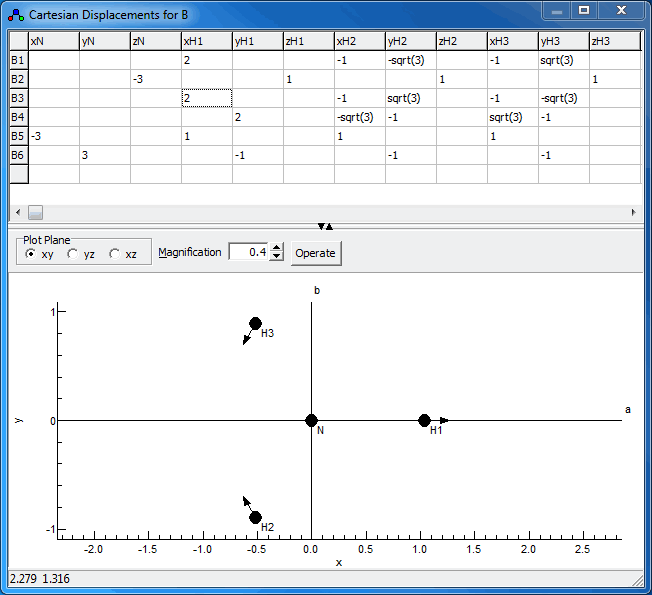### The Controls

The plot at the bottom shows the current internal coordinate, i.e. the selected row in the top grid. The arrows indicate how far each atom moves. The plot updates semi-automatically; use "Operate, Check" to force an update.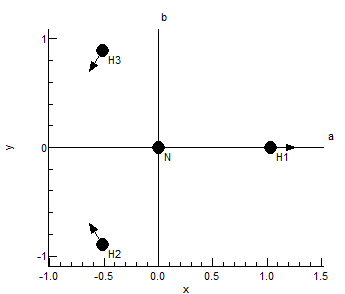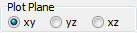The "Plot Plane" control allows the selection of the plane in which to plot the molecule.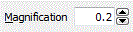The magnification selects the scale factor to use for the arrows.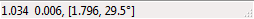The status bar shows the latest mouse position; draw a box with the mouse to measure distance and angles.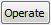The operate button brings up a menu of possible operations:
 Check Check the contents of the top grid, and update the plot to match Remove R, T Force the coordinates to be orthogonal to rotational and translational coordinates by subtracting the projection onto these coordinates. The details of the calculation are displayed in the Log Window. Calculate from l matrix Set the B matrix by calculating it from the l matrix assuming the internal Cartesian coordinates are the same as the normal coordinates. The equation used is: B = lT M1/2

### Cartesian Displacement Coordinates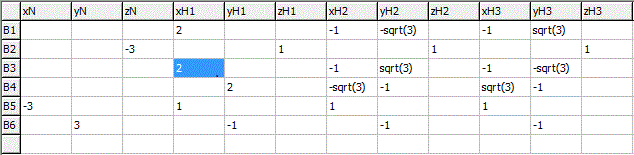The grid at the top allows individual elements of the B, to be entered. The elements can be given as simple numerical values, but can also be given as general expressions involving symbolic values. When reading the matrix, unknown variables are automatically created as parameters to the current Cartesian displacememts object, and they can then be edited in the Constants Window. If dealing with more than one isotopologue, these variables are better placed in a variables object, (with Global set to true) so the same value can be used in all species. Note that the grid will expand automatically as rows are added. The grid should be cleared if the B matrix is to be given using an Internal Coordinates object.

## Cartesian Displacement Settings

 Active Set true to use this internal coordinates object; there must be no more than one active internal coordinates object at a time. InPrincipalAxis Specifies the axis system used in specifying the Cartesian displacements; either the principal axis system or the axis system used in the z matrix specification. Normalize If true, the rows of the matrix given are normalized before use in further calculations